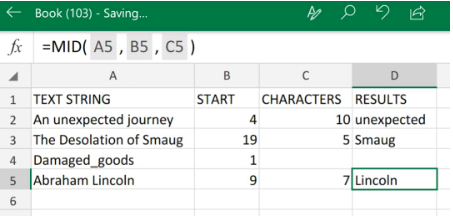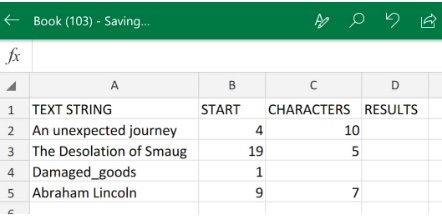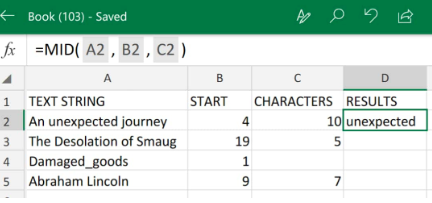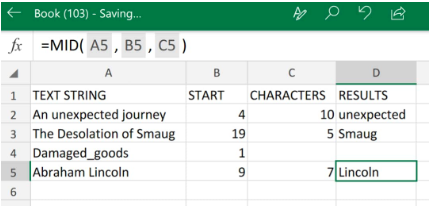Get instant live expert help with Excel or Google Sheets“My Excelchat expert helped me in less than 20 minutes, saving me what would have been 5 hours of work!”

#### Post your problem and you’ll get expert help in seconds.

Your message must be at least 40 characters
Our professional experts are available now. Your privacy is guaranteed.

# How to use the MID function in Excel – Excelchat

The MID Excel function is designed to extract a substring from the middle of any given text string.

The MID function is classified as an Excel text function which returns a specific number of characters beginning at a position of our choosing.Figure 1. of MID Function in Excel

## Generic Formula

`=MID(text,start_num,num_chars)`

• text = The original text string to extract from
• start_num = The exact position of the initial character to extract
• num_chars = The amount of characters to be extracted

## How to Use the Excel Mid Function

Using the following simple steps will help us extract text with the MID function Excel;

1. We must first collect and arrange our text strings for extraction in our worksheet:Figure 2. of Text Strings for Extraction in Excel

Be sure to leave a bank column of cells for the MID formula to return our text results (see column D above).

Our goal here is to extract a specific number of text characters from the text strings in column A.

1. The Excel MID formula we will insert into cell D2 of our spreadsheet example is as follows:

`=MID(A2,B2,C2)`Figure 3. of MID Function in Excel

The MID formula extracted the word “unexpected” (10 characters) from a given position in our original text string.

1. Modify and copy the MID Excel function in cell D2 above, down into the other cells in the column for similar results:Figure 4. MID Function in Excel

## Note

• The MID function will always return a text string of characters, even if our extracted substring contains digits only.
• If our start_num value is more than the length of our original text string , then the Excel Mid formula will return an empty string value  (“”).
• If our start_num value is lower than 1, the Excel Mid formula will return an error #VALUE!
• If our num_chars value is below 0 ( a negative number), the Excel Mid formula will return the error #VALUE!
• If our num_chars value is 0, the Excel Mid formula will return an empty text string (blank cell).

## Instant Connection to an Excel Expert

Most of the time, the problem you will need to solve will be more complex than a simple application of a formula or function. If you want to save hours of research and frustration, try our live Excelchat service! Our Excel Experts are available 24/7 to answer any Excel question you may have. We guarantee a connection within 30 seconds and a customized solution within 20 minutes.